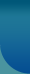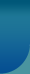Home >> Socio Short Notes >> Bell Curve

# Bell Curve

The bell curve provides a foundation for the statistical procedures in sociology. It is a histogram, but with such fine distinctions between outcomes that it is a line in the shape of a bell. Beneath this curve are all possible outcomes, with the outcomes on the x-axis and the y-axis describing the proportion or probability for each outcome. The ''tails'' of the curve extend indefinitely. The shape is symmetrical and unimodel so that the distribution's mean, median, and mode are identical and in the center of the distribution.

The bell curve can be used for hypothesis testing. The central limit theorem states that, even when individual scores are not normally distributed, in random samples of a sufficient size, the distribution of sample means will be approximately normally distributed around the population mean. Thus, sociologists can examine the probability of producing a specific sample mean, based on a hypothesized population mean. If a sample mean is unlikely to occur based on the hypothesized population mean, the sociologist can reject the hypothesized population mean. Similarly, relationships between variables can be tested by studying how likely it would be to find a specific relationship in a sample if there was no relationship in the population.© 2023 Sociology Guide.Com